Test: Relations & Functions- Case Based Type Questions

# Test: Relations & Functions- Case Based Type Questions

Test Description

## 10 Questions MCQ Test Mathematics (Maths) Class 11 | Test: Relations & Functions- Case Based Type Questions

Test: Relations & Functions- Case Based Type Questions for Commerce 2023 is part of Mathematics (Maths) Class 11 preparation. The Test: Relations & Functions- Case Based Type Questions questions and answers have been prepared according to the Commerce exam syllabus.The Test: Relations & Functions- Case Based Type Questions MCQs are made for Commerce 2023 Exam. Find important definitions, questions, notes, meanings, examples, exercises, MCQs and online tests for Test: Relations & Functions- Case Based Type Questions below.
Solutions of Test: Relations & Functions- Case Based Type Questions questions in English are available as part of our Mathematics (Maths) Class 11 for Commerce & Test: Relations & Functions- Case Based Type Questions solutions in Hindi for Mathematics (Maths) Class 11 course. Download more important topics, notes, lectures and mock test series for Commerce Exam by signing up for free. Attempt Test: Relations & Functions- Case Based Type Questions | 10 questions in 20 minutes | Mock test for Commerce preparation | Free important questions MCQ to study Mathematics (Maths) Class 11 for Commerce Exam | Download free PDF with solutions
 1 Crore+ students have signed up on EduRev. Have you?
Test: Relations & Functions- Case Based Type Questions - Question 1

### A Relation R from A to B can be depicted pictorially using arrow diagram. In arrow diagram, we write down the elements of two sets A and B in two disjoint circles. Then we draw arrow from set A to set B whenever (a, b) ∈ R. An example of information depicted through an arrow diagram is shown below. For example: A company has four categories of employees given by Assistants (A), Clerks (C), Managers (M) and an Executive Officer (E). The company provides ₹10,000, ₹25,000, ₹50,000 and ₹1,00,000 as salaries to the people who work in the categories A, C, M and E respectively. Here A1, A2, A3, A4 and A5 are Assistants; C1, C2, C3, C4 are Clerks; M1, M2, M3 are Managers and E1, E2 are Executive Officers then the relation R is defined by xRy, where x is the salary given to person y.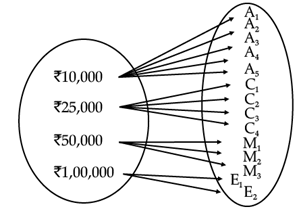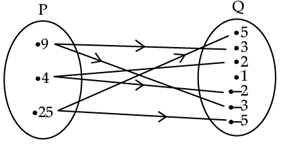The relation defined in the above arrow diagram from set P to set Q is

Detailed Solution for Test: Relations & Functions- Case Based Type Questions - Question 1

When x = 9, y = ±3

When x = 4, y = ±2

When x = 25, y = ±5

∴ x is a square of y.

Test: Relations & Functions- Case Based Type Questions - Question 2

### A Relation R from A to B can be depicted pictorially using arrow diagram. In arrow diagram, we write down the elements of two sets A and B in two disjoint circles. Then we draw arrow from set A to set B whenever (a, b) ∈ R. An example of information depicted through an arrow diagram is shown below. For example: A company has four categories of employees given by Assistants (A), Clerks (C), Managers (M) and an Executive Officer (E). The company provides ₹10,000, ₹25,000, ₹50,000 and ₹1,00,000 as salaries to the people who work in the categories A, C, M and E respectively. Here A1, A2, A3, A4 and A5 are Assistants; C1, C2, C3, C4 are Clerks; M1, M2, M3 are Managers and E1, E2 are Executive Officers then the relation R is defined by xRy, where x is the salary given to person y.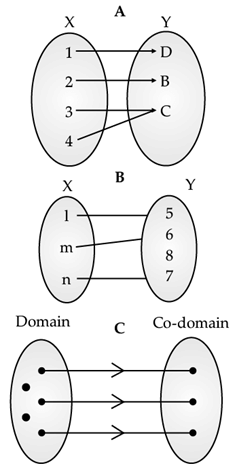Which among the above figures shows a Relation between the two non - empty sets?

Detailed Solution for Test: Relations & Functions- Case Based Type Questions - Question 2

Figures A and B show relations. Figure C shows a function but not a relation.

Test: Relations & Functions- Case Based Type Questions - Question 3

### A Relation R from A to B can be depicted pictorially using arrow diagram. In arrow diagram, we write down the elements of two sets A and B in two disjoint circles. Then we draw arrow from set A to set B whenever (a, b) ∈ R. An example of information depicted through an arrow diagram is shown below. For example: A company has four categories of employees given by Assistants (A), Clerks (C), Managers (M) and an Executive Officer (E). The company provides ₹10,000, ₹25,000, ₹50,000 and ₹1,00,000 as salaries to the people who work in the categories A, C, M and E respectively. Here A1, A2, A3, A4 and A5 are Assistants; C1, C2, C3, C4 are Clerks; M1, M2, M3 are Managers and E1, E2 are Executive Officers then the relation R is defined by xRy, where x is the salary given to person y.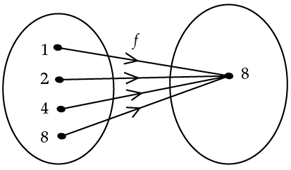The relation defined in the above arrow diagram from set A to set B is

Detailed Solution for Test: Relations & Functions- Case Based Type Questions - Question 3

1,2,4 and 8 are factors of 8.

Test: Relations & Functions- Case Based Type Questions - Question 4

A Relation R from A to B can be depicted pictorially using arrow diagram. In arrow diagram, we write down the elements of two sets A and B in two disjoint circles. Then we draw arrow from set A to set B whenever (a, b) ∈ R. An example of information depicted through an arrow diagram is shown below. For example:

A company has four categories of employees given by Assistants (A), Clerks (C), Managers (M) and an Executive Officer (E). The company provides ₹10,000, ₹25,000, ₹50,000 and ₹1,00,000 as salaries to the people who work in the categories A, C, M and E respectively. Here A1, A2, A3, A4 and A5 are Assistants; C1, C2, C3, C4 are Clerks; M1, M2, M3 are Managers and E1, E2 are Executive Officers then the relation R is defined by xRy, where x is the salary given to person y.If the number of elements in set A and set B are p and q, then the number of relations from A to B are

Detailed Solution for Test: Relations & Functions- Case Based Type Questions - Question 4 Number of relations from A to B is 2n(A)n(B) =2pq.
Test: Relations & Functions- Case Based Type Questions - Question 5

A Relation R from A to B can be depicted pictorially using arrow diagram. In arrow diagram, we write down the elements of two sets A and B in two disjoint circles. Then we draw arrow from set A to set B whenever (a, b) ∈ R. An example of information depicted through an arrow diagram is shown below. For example:

A company has four categories of employees given by Assistants (A), Clerks (C), Managers (M) and an Executive Officer (E). The company provides ₹10,000, ₹25,000, ₹50,000 and ₹1,00,000 as salaries to the people who work in the categories A, C, M and E respectively. Here A1, A2, A3, A4 and A5 are Assistants; C1, C2, C3, C4 are Clerks; M1, M2, M3 are Managers and E1, E2 are Executive Officers then the relation R is defined by xRy, where x is the salary given to person y.If the number of elements in set A and set B are p and q then the number of functions from A to B are

Detailed Solution for Test: Relations & Functions- Case Based Type Questions - Question 5 Number of functions from A to B n(B)n(A) = qp
Test: Relations & Functions- Case Based Type Questions - Question 6

Consider the graphs of the functions f(x), h(x) and g(x).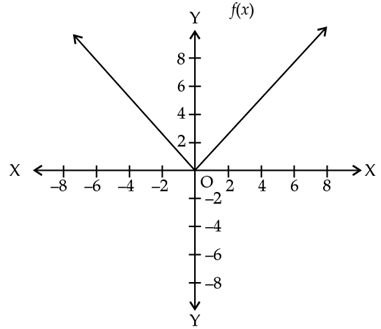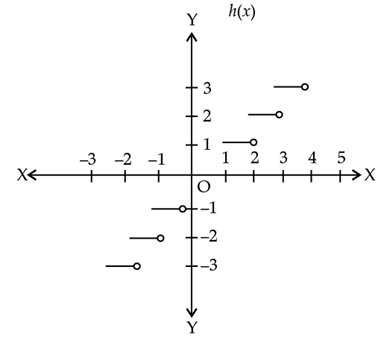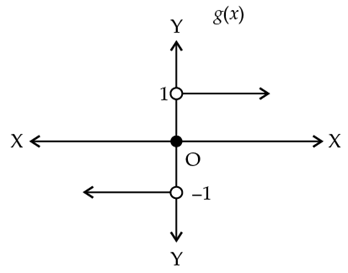The domain of f(x) is ..................

Detailed Solution for Test: Relations & Functions- Case Based Type Questions - Question 6

f(x) = |x|. The domain of f(x) is R.

Test: Relations & Functions- Case Based Type Questions - Question 7

Consider the graphs of the functions f(x), h(x) and g(x).The range of g(x) is ................

Detailed Solution for Test: Relations & Functions- Case Based Type Questions - Question 7

g(x) is the signum function. Its range is {–1, 0, 1}.

Test: Relations & Functions- Case Based Type Questions - Question 8

Consider the graphs of the functions f(x), h(x) and g(x).The range of h(x) is .......................

Detailed Solution for Test: Relations & Functions- Case Based Type Questions - Question 8

h(x) = [x] is the greatest integer function. Its range is Z (set of integers)

Test: Relations & Functions- Case Based Type Questions - Question 9

Consider the graphs of the functions f(x), h(x) and g(x).The value of h(–2.3) is ...................

Detailed Solution for Test: Relations & Functions- Case Based Type Questions - Question 9

h(–2.3) = [–2.3] = –3

Test: Relations & Functions- Case Based Type Questions - Question 10

Consider the graphs of the functions f(x), h(x) and g(x).The value of f(10) is ...................

Detailed Solution for Test: Relations & Functions- Case Based Type Questions - Question 10

Since 10 > 0, f(10) = 1.

## Mathematics (Maths) Class 11

156 videos|176 docs|132 tests
Information about Test: Relations & Functions- Case Based Type Questions Page
In this test you can find the Exam questions for Test: Relations & Functions- Case Based Type Questions solved & explained in the simplest way possible. Besides giving Questions and answers for Test: Relations & Functions- Case Based Type Questions, EduRev gives you an ample number of Online tests for practice

## Mathematics (Maths) Class 11

156 videos|176 docs|132 tests(Scan QR code)